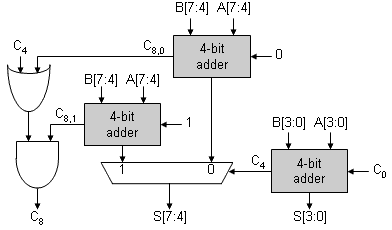Fdis

In thinking about the propagation delay of a ripple-carry adder, we see that the higher-order bits are "waiting" for their carry-ins to propagate up from the lower-order bits. Suppose we split off the high-order bits and create two separate adders: one assuming that the carry-in was 0 and the other assuming the carry-in was 1. Then when the correct carry-in was available from the low-order bits, it could be used to select which high-order sum to use. The diagram below shows this strategy applied to an 8-bit adder:1. Compare the latency of the 8-bit carry-select adder show above to a regular 8-bit ripple-carry adder.
2. The logic shown for C8 seems a bit odd. One might have expected C8 = C8,0 + (C4*C8,1). Explain why both implementations are equivalent and suggest why the logic shown above might be prefered. Hint: What can we say about C8,1 when C8,0=1?
Solution:

1. For the low-order 4 bits, the latency is the same for both implementations: TPD,4-BIT ADDER. But with the carry-select adder, the remaining latency is the propagation delay of the 4-bit 2:1 multiplexer (TPD,2:1 MUX) instead of the longer time it takes for the carry to ripple through another 4 bits of adder (TPD,4-BIT ADDER). If we consider an N-bit adder, the latencies are:
TPD,N-BIT RIPPLE = 2 * TPD,(N/2)-BIT RIPPLE
TPD,N-BIT CARRY-SELECT = TPD,(N/2)-BIT RIPPLE + TPD,2:1 MUX
As N gets large the carry-select adder is almost twice as fast as a ripple-carry adder since the delay through the 2:1 mux is independent of N. The carry-select strategy can be applied recursively to the (N/2)-bit ripple-carry adder, and so on, ultimately producing an adder with O(log2N) latency.

2. If we think about carry generation, it's easy to see that if C8,0=1 then C8,1=1, i.e., if we get a carry with CIN=0, we'll also get a carry when CIN=1. Using this fact we can do a little Boolean algebra:
`C8 = C8,0 + (C4 * C8,1)____= C8,0*(C8,1 + C8,1) + (C4 * C8,1)____= (C8,0 * C8,1) + (C8,0 * C8,1) + (C4 * C8,1)= (C8,0 * C8,1) + 0 + (C4 * C8,1)= (C8,0 + C4)*C8,1`
In the worst case (the carry-in rippling all the way up), C8,1 will take a little longer to compute than C8,0, so the logic for C8 shown in the diagram will be a little faster since it provides the shorter path to C8.